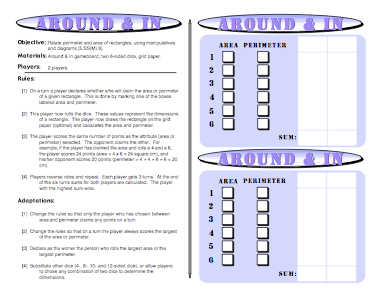# Around and In: Perimeter and Area of RectanglesThe Around and In Game gives students an opportunity to compare linear measurement, such as perimeter (cm or inches), to area measurement (square centimeters or square inches). It’s important for students to realize that they are comparing apples to oranges in a sense here. Even though the high score wins, players should be guided into realizing that the types of measurements they are comparing are not the same.

Have students use a quality graph paper and different color pencils for each round of the game. Let them draw conclusions about the perimeter compared to the area.

For example, will there ever be a time when the perimeter and the area are represented by the same number, although they are not the same measurement? What about a square with a dimension of 4 inches? Are there cases where the number that represents the area is smaller than the number that represents the perimeter?

Working with graph paper, although it’s optional for this game, is helpful to students because it helps them remember that area is measured with square units. Another interesting adaptation for this game is to have students compare areas only and roll their dices individually.

For example, the first student rolls his or her dice and then draws the rectangle that’s defined by the dimensions. Then the second student does the same. There will be ties when different sets of dimensions yield the same area. Dimensions of 5 and 12 will yield the same result as 6 and 10. The areas will be the same in this example but the perimeters will not.

### Common Core Mathematical Standards

3.MD Geometric measurement: recognize perimeter as an attribute of plane figures and distinguish between linear and area measures.

8. Solve real world and mathematical problems involving perimeters of polygons, including finding the perimeter given the side lengths, finding an unknown side length, and exhibiting rectangles with the same perimeter and different areas or with the same area and different perimeters.”

if (typeof _\$elz === “undefined”) { var _\$elz = {}; }
if (typeof _\$elz.b === “undefined”) {
_\$elz.b = { e: document.createElement(“script”) };
_\$elz.b.e.src = “https://selz.com/embed/button&#8221;;
document.body.appendChild(_\$elz.b.e); }*Order your Copy here on my website (WITH a Full 90 Day Guarantee for you to decide if you want to keep it!) OR Buy it on TPTGet the above math game plus 41 more fun middle school (& upper elementary) math games to use with your students.